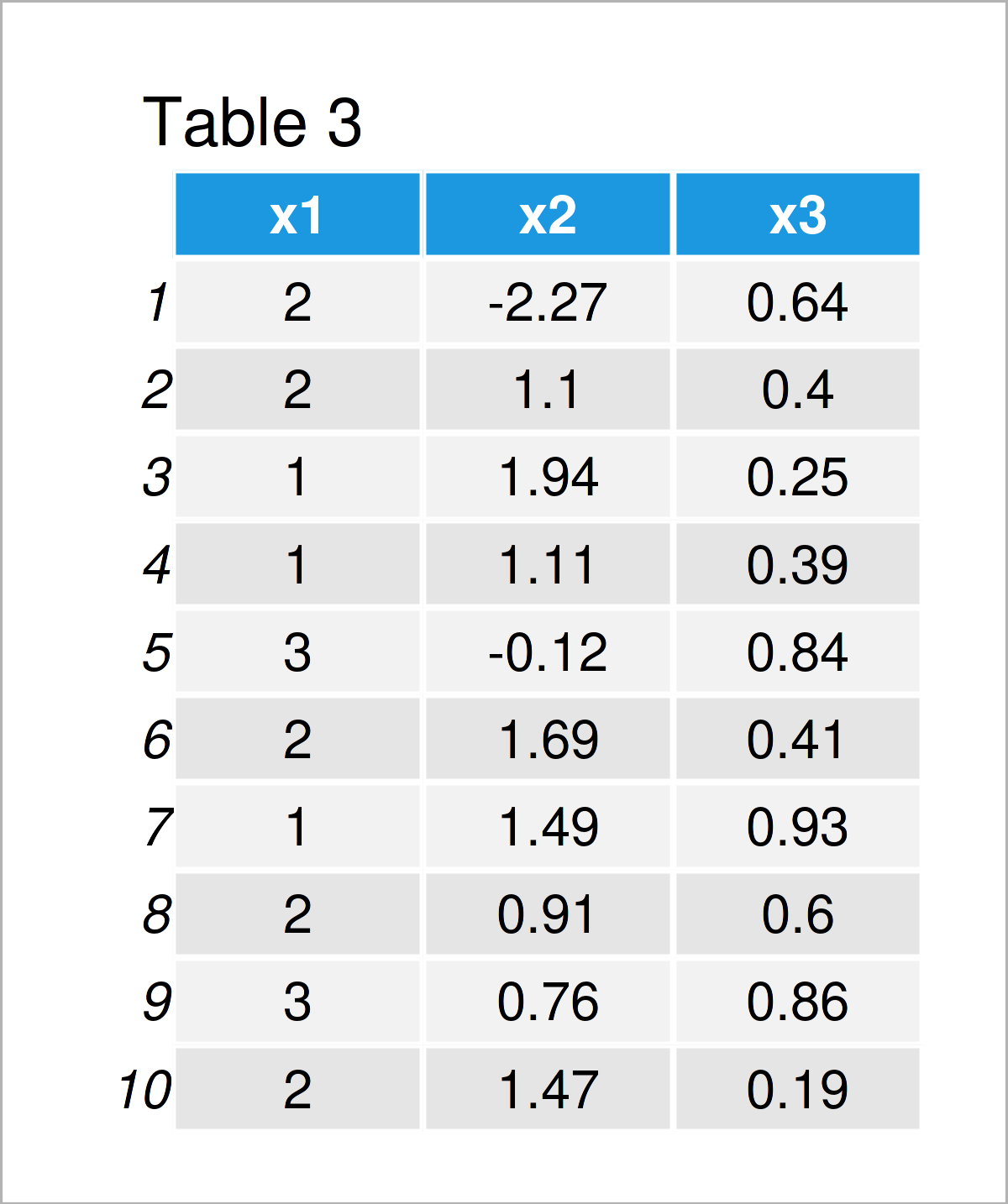# R Error in colMeans(x, na.rm = TRUE) : ‘x’ must be numeric (3 Examples)

This tutorial shows how to debug the “Error in colMeans(x, na.rm = TRUE) : ‘x’ must be numeric” in the R programming language.

The post is structured as follows:

Let’s dive into it:

## Creating Example Data

Let’s first construct some exemplifying data:

```set.seed(67932)                                      # Create example data frame
data <- data.frame(x1 = sample(LETTERS[1:3], 10, replace = TRUE),
x2 = round(rnorm(10), 2),
x3 = round(runif(10), 2))
data                                                 # Print example data frame```As you can see based on Table 1, our example data is a data frame and contains ten rows and three columns.

## Example 1: Reproduce the Error in colMeans(x, na.rm = TRUE) : ‘x’ must be numeric

Example 1 explains how to replicate the “Error in colMeans(x, na.rm = TRUE) : ‘x’ must be numeric”.

Let’s assume that we want to apply a Principal Component Analysis based on these data.

Then, we might try to apply the prcomp function to our data as shown below:

```prcomp(data)                                         # Try to apply prcomp function
# Error in colMeans(x, na.rm = TRUE) : 'x' must be numeric```

Unfortunately, the “Error in colMeans(x, na.rm = TRUE) : ‘x’ must be numeric” is returned.

The reason for this error message is that our data frame contains the column x1 which has the character class (the same error would appear in case of factor columns).

So how could we fix that? There are basically two alternatives, and I’m going to explain these alternatives in the following examples.

## Example 2: Fix the Error by Removing Non-Numeric Columns

In this example, I’ll demonstrate how to drop all non-numeric variables from a data frame to avoid the “Error in colMeans(x, na.rm = TRUE) : ‘x’ must be numeric”.

We can use the unlist, lapply, and is.numeric functions to create such a data frame subset:

```data_new1 <- data[ , unlist(lapply(data,             # Remove non-numeric columns
is.numeric))]
data_new1                                            # Print updated data frame```As shown in Table 2, we have created a new data frame with the previous R syntax. This data frame contains only the two numeric columns x2 and x3.

Next, we can apply the prcomp function to these data:

```prcomp(data_new1)                                    # Apply prcomp function
# Standard deviations (1, .., p=2):
#  1.2283189 0.2428404
#
# Rotation (n x k) = (2 x 2):
#            PC1        PC2
# x2  0.99647810 0.08385344
# x3 -0.08385344 0.99647810```

Works fine!

## Example 3: Fix the Error by Converting Non-Numeric Columns to Numbers

Example 3 demonstrates how to convert non-numeric categorical data to numeric data in order to get rid of the “Error in colMeans(x, na.rm = TRUE) : ‘x’ must be numeric”.

To accomplish this, we have to apply the as.numeric and as.factor functions to our non-numeric data frame column x1:

```data_new2 <- data                                    # Duplicate data frame
data_new2\$x1 <- as.numeric(as.factor(data_new2\$x1))  # Convert categories to numbers
data_new2                                            # Print updated data frame```Table 3 shows the output of the previous code: We have transformed the categorical variable x1 into numbers.

Now, we can apply the prcomp function without any problems:

```prcomp(data_new2)                                    # Apply prcomp function
# Standard deviations (1, .., p=3):
#  1.2734878 0.6608866 0.2316053
#
# Rotation (n x k) = (3 x 3):
#           PC1       PC2         PC3
# x1 -0.3082818 0.9444851 -0.11362327
# x2  0.9471298 0.3158997  0.05614757
# x3 -0.0889241 0.0903067  0.99193609```

## Video & Further Resources

Have a look at the following video on my YouTube channel. In the video, I’m showing the content of this tutorial.

Furthermore, you may read the related tutorials on my website. A selection of articles can be found here.

Summary: You have learned in this article how to avoid the “Error in colMeans(x, na.rm = TRUE) : ‘x’ must be numeric” in R programming. Let me know in the comments section below, in case you have further questions.

Subscribe to the Statistics Globe Newsletter